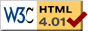### Journée-séminaire de combinatoire

#### (équipe CALIN du LIPN, université Paris-Nord, Villetaneuse)

Le 05 novembre 2019 à 14h00 en B107, Vonjy Rasendrahasina nous parlera de : On the sparse random acyclic digraphs

Résumé : In this talk, we consider the probability space of random digraphs defined as follow. We generate a random undirected graph by taking the classical Erdős–Rényi model $\mathbb G(n,p)$. Thereafter, each edge is given a direction, where each of the two directions has probability $\frac{1}{2}$ and all choices are made independently. The result is a simple digraph on $n$ vertices. We will study the the probability that a random digraph is acyclic when $p=O(1/n)$, i.e. it does not contain a directed cycle when the number of edges is linear in the number of vertices. This is a joint work with Dimbinaina Ralaivaosaona and Stephan Wagner.

 Dernière modification : Monday 24 January 2022Contact pour cette page : Cyril.Banderier at lipn.univ-paris13.fr